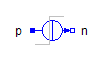Impedance - MapleSim Help

Quasistationary Multiphase Impedance

Multiphase linear impedanceDescription The Quasistationary Multiphase Impedance (or Impedance) component contains $m$ single-phase impedance models, each connected between corresponding phases of ${\mathrm{plug}}_{p}$ and ${\mathrm{plug}}_{n}$.Connections

 Name Description Modelica ID ${\mathrm{plug}}_{p}$ Positive quasistationary multiphase plug plug_p ${\mathrm{plug}}_{n}$ Negative quasistationary multiphase plug plug_n $\mathrm{Heat Port}$ heatPortParameters

 Name Default Units Description Modelica ID $m$ $3$ Number of phases m ${Z}_{\mathrm{ref}}$ Complex impedances Z_ref ${T}_{\mathrm{ref}}$ $\mathrm{fill}\left(293.15,m\right)$ $K$ Reference temperatures T_ref ${\mathrm{\alpha }}_{\mathrm{ref}}$ $\mathrm{zeros}\left(m\right)$ $\frac{1}{K}$ Temperature coefficient of resistance alpha_ref Use Heat Port $\mathrm{false}$ True (checked) means heat port is enabled useHeatPort $T$ ${T}_{\mathrm{ref}}$ $K$ Fixed device temperatures if useHeatPort = false T Frequency dependent $\mathrm{false}$ Consider frequency dependency, if true frequencyDependent ${f}_{\mathrm{ref}}$ $1$ $\mathrm{Hz}$ Relative frequency, if frequency dependency is considered f_refModelica Standard Library The component described in this topic is from the Modelica Standard Library. To view the original documentation, which includes author and copyright information, click here.